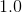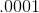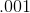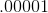# Algebra 1 : How to find decimal equivalent to a percentage

## Example Questions

### Example Question #41 : How To Find Decimal Equivalent To A Percentage

If you owe the bankof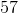dollars, how much money do you owe them?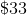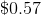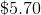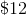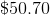Explanation:

To solve this problem, first, you must convert the percentage above into a decimal.

To find the decimal equivalent of a percentage, simply move the decimal (if there is none, remember that there is always an implied decimal after the one's digit) to the left twice. In other words divide the percentage by one hundred.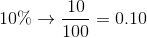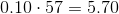### Example Question #42 : How To Find Decimal Equivalent To A Percentage

If you owe the bank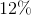ofdollars, how much money do you owe them?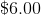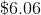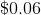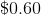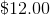Explanation:

To solve this problem, first, you must convert the percentage above into a decimal. To find the decimal equivalent of a percentage, simply move the decimal (if there is none, remember that there is always an implied decimal after the one's digit) to the left twice. In other words, divide the percentage by one hundred.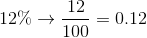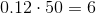### Example Question #43 : How To Find Decimal Equivalent To A Percentage

Write the following percentage as a decimal.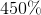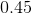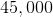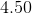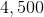Explanation:

Write the following percentage as a decimal.To write a percentage as a decimal, we simply need to move the decimal place two spaces to the left.

Thus,Becomes,### Example Question #41 : How To Find Decimal Equivalent To A Percentage

Find the decimal of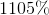.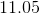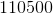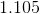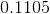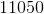Explanation:

The number in percent is out of 100 parts.  Remove the percentage sign and divide this number by 100.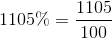This is the same as moving the decimal place of 1105 two places to the left.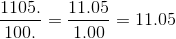The answer is:### Example Question #45 : How To Find Decimal Equivalent To A Percentage

Find the decimal of.Explanation:

The number in percent is out of 100 parts.  Remove the percentage sign and divide this number by 100.This is the same as moving the decimal place of 1105 two places to the left.The answer is:### Example Question #41 : How To Find Decimal Equivalent To A Percentage

Rewrite the following percent as a decimal: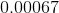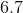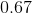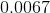Explanation:

Rewrite the following percent as a decimal: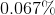To convert between decimals and percents, we simply need to move the decimal point.

When going from a decimal to a percent, move the point two spaces to the right.

When going from a percent to a decimal, move the point two spaces to the left.

So this,Becomes### Example Question #47 : How To Find Decimal Equivalent To A Percentage

Which of the following is the decimal equivalent of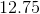%?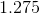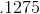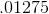Explanation:

To find the decimal equivalent of a percentage, simply move the decimal (if there is none, remember that there is always an implied decimal after the one's digit) to the left twice.  The best answer is:### Example Question #48 : How To Find Decimal Equivalent To A Percentage

Which of the following is the decimal equivalent of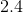%?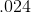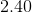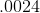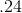Explanation:

To find the decimal equivalent of a percentage, simply move the decimal (if there is none, remember that there is always an implied decimal after the one's digit) to the left twice.  The best answer is:### Example Question #49 : How To Find Decimal Equivalent To A Percentage

Which of the following is the decimal equivalent of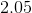%?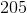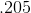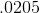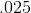Explanation:

To find the decimal equivalent of a percentage, simply move the decimal (if there is none, remember that there is always an implied decimal after the one's digit) to the left twice.  The best answer is:### Example Question #50 : How To Find Decimal Equivalent To A Percentage

Which of the following is the decimal equivalent of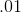%?